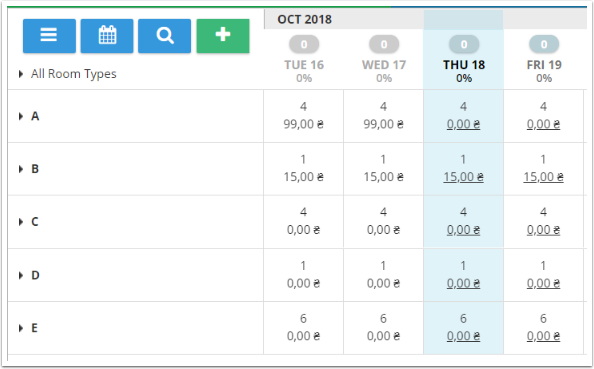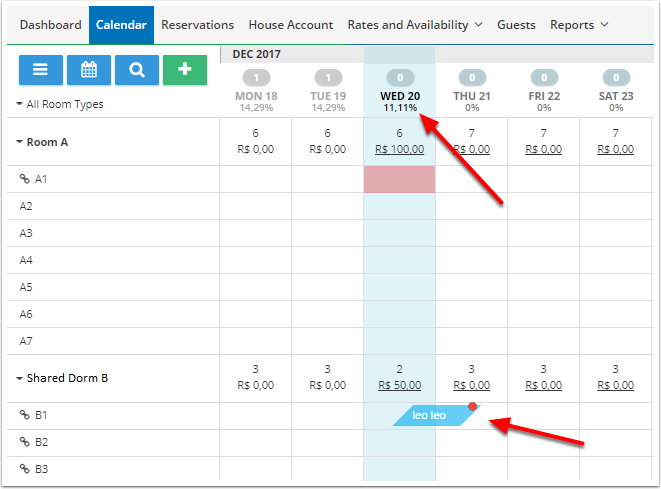# Shared Inventory - Occupancy and Availability Calculation

Follow

The total number of rooms used for calculating occupancy can vary based on how the rooms are linked using shared inventory. Instead of adding all the existing rooms together, the system will only use one of the rooms when calculating availability percentage on the calendar or in the reports, as these rooms are the same physical space but for sale in different layouts.

In the example below, we see room category A and room category B (dorm)

Room category A has 7 rooms and room category B has 3 rooms.

Room A1 is linked to rooms B1, B2 and B3.The screenshot below shows how the shared inventory is set up for this example:Despite ten rooms being listed on the calendar ( 7 A rooms+ 3 B rooms = 10), we don't really have ten rooms and cannot use ten to calculate the occupancy.

If all of rooms in Type A rooms are booked, I cannot sell any rooms of Room Type B, therefore my maximum room quantity is 7.

If 1 of the rooms in Type B are booked, I cannot sell Room Type A(1). Therefore the maximum room quantity is 9 (6 A rooms and 3 B rooms).

If neither room types are occupied, the system will take a number of rooms from primary accommodation type. In this example, the system would use 7.

Please note: The calculations are taking into consideration that no reservations, blocks or holds exist.

IMPORTANT: primary accommodation in shared inventory should always be the real physical accommodation

Room types:

• 5 Room types: A(4 rooms), B(1 room), C(4 rooms), D(1 room), E (6 rooms)

Links:

• A (primary) -> B (secondary)
• D (primary) -> C (secondary)Case 1: Reservation for A (primary)

A: 4 rooms

B: 0 rooms

C: 0 rooms

D: 1 room

E: 6 rooms

Occupancy = 1 / (4 + 1 + 6) = 9.09Case 2: Reservation for B (secondary)

A: 0 rooms

B: 1 room

C: 0 rooms

D: 1 room

E: 6 rooms

Occupancy = 1 / (1 + 1 + 6) = 12.5Case 3: No reservations

A: 4 rooms

B: 0 rooms

C: 0 rooms

D: 1 room

E: 6 rooms

Occupancy = 0 / (4 + 1 + 6) = 0In the following example, we can see a reservation was made for room A1 which is linked to all three rooms in category B.

In this case, the system will consider the occupancy based on the 7 A rooms because only 7 total reservations will be possible.

Therefore, 7 rooms equals 100% availability and 1 room equals 14.29% of the availability. As one room is reserved, this becomes 14.29% occupancy, as is shown on the calendar below.In the case that we have a reservation in category B, the property will have 9 rooms available to be reserved in total for this date (6 remaining A rooms, 2 remaining B rooms and 1 reserved B room).

Therefor, 9 rooms equals 100% availability and one room equals 11.11% of the availability. As one room is reserved, this becomes 11.11% occupancy as is shown on the calendar below.Was this article helpful?
0 out of 0 found this helpful
Have more questions? Contact Support